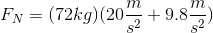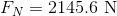# AP Physics B : Calculating Weight

## Example Questions

### Example Question #11 : Ap Physics 1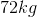astronaut on Earth uses a space elevator to get to a space station. From the ground, the elevator accelerates upwards at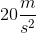. During this acceleration, what is the normal force acting on the astronaut?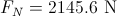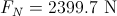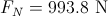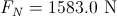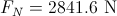Explanation:

This question essentially asks how much the astronaut will weigh during acceleration. The two forces acting on the astronaut are the downward gravitational force and the upward normal force. The net force acting on this astronaut is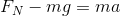.

Notice that the net force is set to equal to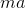to show that the astronaut is accelerating due to the elevator.

Isolate the normal force.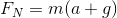Use the given values to solve.